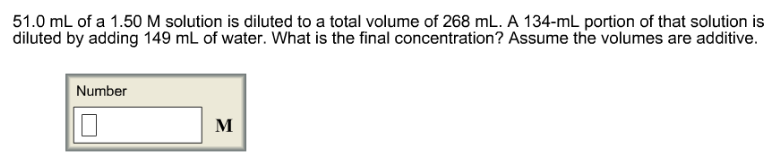# Problem: 51.0 mL of a 1.50 M solution is diluted to a total volume of 268 mL. A 134-mL portion of that solution is diluted by adding 149 mL of water. What is the final concentration? Assume the volumes are additive.

###### FREE Expert Solution
82% (439 ratings)###### Problem Details

51.0 mL of a 1.50 M solution is diluted to a total volume of 268 mL. A 134-mL portion of that solution is diluted by adding 149 mL of water. What is the final concentration? Assume the volumes are additive.